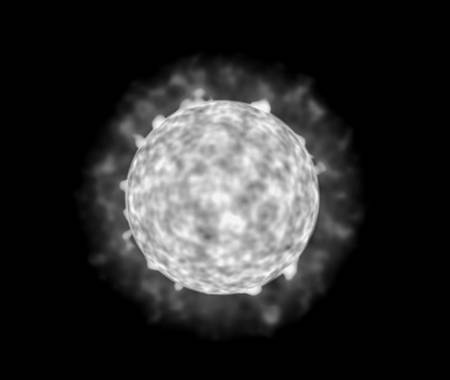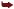# Animation

Animation, whether it's vertex- or skeleton-based, is a CPU-hungry process. If we go the vertex path, we will need interpolations galore to make sure movements are smooth and we can store as few frames as possible. On the skeletal side, quaternion interpolations are just part of the problem. The real meat comes when we need to perform point-matrix multiplies, possibly blending different matrices per vertex to perform multiple bone influences.

Thus, it is not surprising that animating characters is one of the tasks shading languages can be devoted to. All will be achieved through vertex shaders, which will implement the animation algorithm of your choice.

In this example, you could implement keyframe interpolation using a vertex shader. The core interpolator is no big issue. Linear interpolation can be implemented with the following equation:

` Position=positionA*(1-alpha) + positionB*alpha `

where positionA and positionB are the two position endpoints and alpha is the interpolator. This transforms nicely into Cg code with the lerp function, which implements a linear interpolation:

` Position=lerp(positionA, positionB, alpha); `

The only problem with this approach is how to pass both positions to the vertex shader, which, if you recall, must act like a filter: receiving one set of vertices and transforming it. Currently, there is no way to pass both meshes, so we need some tricks to get this working. The trick we will use is to store the second mesh as texturing coordinates, so we can access them from within the shader. Specifically, we will pass a position, the regular float2 set of texture coordinates, one color (float4), and a second set of texture coordinates (float3), which will hold the second position to interpolate. Take a look at the following code:

` void keyframe (float3 positionA: POSITION, float3 positionB: TEXCOORD1, float4 color: COLOR, float2 texcoord: TEXCOORD0, out float4 oPosition: POSITION, out float2 oTexCoord: TEXCOORD0, out float3 oColor: COLOR, uniform float alpha, uniform float4x4 modelviewProj) { float3 position=lerp(positionA, positionB, alpha); oPosition=mul(modelviewProj,float4(position,1)); oTexCoord=texCoord; oColor=color; } `

That's just four lines really, and it saves developers a lot of headaches. All we need to do is compute the alpha value based on the distance between both key frames. Notice that the preceding code can handle both regular and unevenly spaced keys. We could enhance the code to pass extra data and drive a quadratic or cubic interpolator, but for most uses, linear is fine.

This approach can be adapted easily for facial animation using morphs. After all, we are just interpolating several facial expressions per vertex, so the preceding code, in all its simplicity, is definitely worth checking out. But other, more serious animation problems lie ahead. Wouldn't it be cool to implement a similar approach to skeletal animation? This is a much trickier case because the algorithm is more complex. Recall that skeletal animation (I'm talking about the multibone approach) is resolved in two phases. We first propagate matrices and store, for each vertex, the bones, and thus the matrices that affect it. We also store matrix weights, so we can blend them properly. As you can imagine, this is the cheap stage because the next one implies performing many point-matrix multiplies and blending the results together. Thus, it is the second phase what we would need to improve by coding the actual skinning code into a shader.

Now our animation code will need to receive, along with the vertex we are processing, the matrices that affect it and the corresponding weights. For simplicity, we will restrict the number of bones that influence a vertex to four, so we need to pass four matrices:

` void skinning(float3 position    : POSITION, float3 normal      : NORMAL, float2 texCoord    : TEXCOORD0, float4 weight      : TEXCOORD1, float4 matrixIndex : TEXCOORD2, out float4 oPosition : POSITION, out float2 oTexCoord : TEXCOORD0, out float4 color     : COLOR, uniform Light light, uniform float4    boneMatrix, // 24 matrices uniform float4x4  modelViewProj) { float3 netPosition = 0, netNormal = 0; for (int i=0; i<4; i++)    {    float index = matrixIndex[i];    float3x4 model = float3x4(boneMatrix[index+0], boneMatrix[index+1],                                       boneMatrix[index+2]);    float3 bonePosition = mul(model, float4(position, 1));    float3x3 rotate = float3x3(model.xyz, model.xyz,    model.xyz);    float3 boneNormal = mul(rotate, normal);    netPosition += weight[i] * bonePosition;    netNormal   += weight[i] * boneNormal;    } netNormal = normalize(netNormal); oPosition = mul(modelViewProj, float4(netPosition, 1)); oTexCoord = texCoord; ocolor = Illuminate(light, netPosition, netNormal); } `

We then loop four times (one per matrix), accumulating each matrix contribution to the vertex and normal value. Notice how the call to Illuminate (which is not included for simplicity) computes shading based on these values. Then, for each matrix, the TEXCOORD1 is used to specify its weight, and the TEXCOORD2 is used to index it into a larger bonematrix array. The model variable holds the current bone influence matrix, which is then applied to the vertex and finally to the normal. Then, this bonePosition (the vertex position according to this bone) and boneNormal (the normal according to that same bone) are added, integrating weight on the equation. The equation is exactly the same as the one we used in our discussion on skeletal animation.

#### Procedural Texturing

One of the main uses for shaders in the movie industry is the creation of procedural textures. Here materials are not defined in terms of bitmaps but in mathematical functions that, when combined, produce aesthetically pleasing results. As a simple example, imagine a checkers pattern, each tile being one meter across. Here is the pseudocode for a shader that would compute this on the GPU, thus saving texture and CPU resources:

` checkers (point pos) if (trunc(pos.x) + trunc(pos.y)) is odd       color=black else color=white `

As you can see, procedural textures are always implemented with pixel shaders, where the shader receives the point to texturize and computes output colors in return.

A good library of mathematical functions is essential for procedural textures to work. Most effects are achieved with operators like trigonometric functions, logarithms, and especially, controlled noise functions such as Perlin Noise (for more on this funcion, see the Perlin article listed in Appendix E). This function generates pseudorandom values over a 3D space, so values close in parameter space are close in their noise value; and faraway parameters yield uncorrelated outputs. Think of it as a band-limited, continuous and derivable noise.

Using Perlin Noise properly, you can implement most natural-looking materials, like wood, marble, or clouds. As an example that shows its capabilities, take a look at Figure 21.7, which was taken from a shader by Pere Fort. Here we are using Perlin Noise to compute an animated sun complete with coronas, surface explosions, and animation. The sun is really a quad, textured using a pixel shader.

##### Figure 21.7. Flaming sun disk implemented as a quad with procedural Perlin textures on a pixel shader.Here is the commented source code for this interesting effect, which somehow shows how real-time shaders can actually create some never-before seen effects in computer games. The source code is very lengthy, but I think it provides a clear vision of what the future holds for shading languages:

` struct vp2fp    {    float4 Position : POSITION;    float4 TexCoord : TEXCOORD0;    float4 Param    : TEXCOORD1;    float4 Color    : COLOR0;    }; // INPUTS // TexCoord : TEXCOORD0 = here we encode the actual coordinates plus the time // Param    : TEXCOORD1 = here we encode in x and y the angles to rotate the sun float4 main(vp2fp IN, const uniform sampler2D permTx, const uniform sampler1D gradTx   ):COLOR { //distance vector from center float2 d = IN.TexCoord.xy - float2(0.5f,0.5f); //distance scalar float dist = sqrt(dot(d,d)) * 2; // Sun's radius float radSun = 0.6f; // Halo's radius float radHalo = 1.0f; float3 vCoord = IN.TexCoord.xyz; float freq; if(dist < radSun) // fragment inside the Sun    {    // freq inside the Sun is higher than outside    freq = 64;    // texture coords displacement in 2D    vCoord.xy -= IN.Param.xy;    // sphere function, with 70% depth    vCoord.xy -= d*sqrt(radSun*radSun-dist*dist)*0.7f;    } else //fragment outside the Sun    {    // freq outside the Sun is half than inside    freq = 32;    // a little displacement (20%) of the halo while rotating the sphere    vCoord.xy -= IN.Param.xy*0.2;    } // sum of two octaves of perlin noise, second with half the freq of the first float4 total = 0f.xxxx; total += (perlinNoise3_2D(vCoord*freq  , permTx, gradTx) + 0.7).xxxx*0.33f; total += (perlinNoise3_2D(vCoord*freq/2, permTx, gradTx) + 0.7).xxxx*0.66f; // after getting the result of the noise we can compute the radius of the explosions // we can use one dimension from the result to get a single dimension perlin noise float radExpl = (1 + total.x) * radSun * 0.6f; // this generates a little halo between Sun and explosions, and avoids some aliasing if(radExpl < radSun*1.01f) radExpl = radSun*1.01f; // filtering the colour of fragments // first smoothstep makes explosions darker as the radius increases // second smoothstep makes the halo darker as it becomes far from the sun dist = smoothstep(radExpl,radSun,dist)*0.5f + smoothstep(radHalo,radExpl,dist) * 0.5f; // transform black into red // blue to 0 means obtaining yellow // maintain the alpha input total.rba = float3(total.x*1.8f,0.0f,IN.Color.a); // ensure the ranges of the colors after all modifications total.xyz = clamp(total.xyz,0,1); // return all the modifications with the filter applied return total*dist; } `Core Techniques and Algorithms in Game Programming2003
ISBN: N/A
EAN: N/A
Year: 2004
Pages: 261